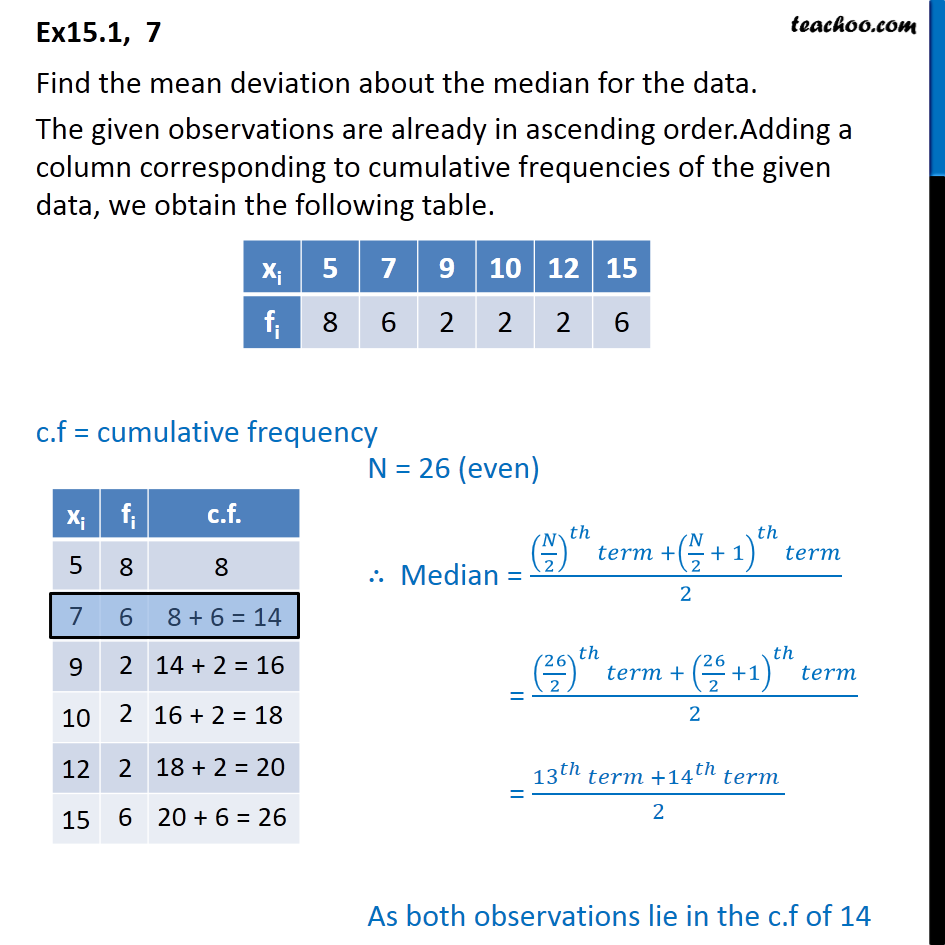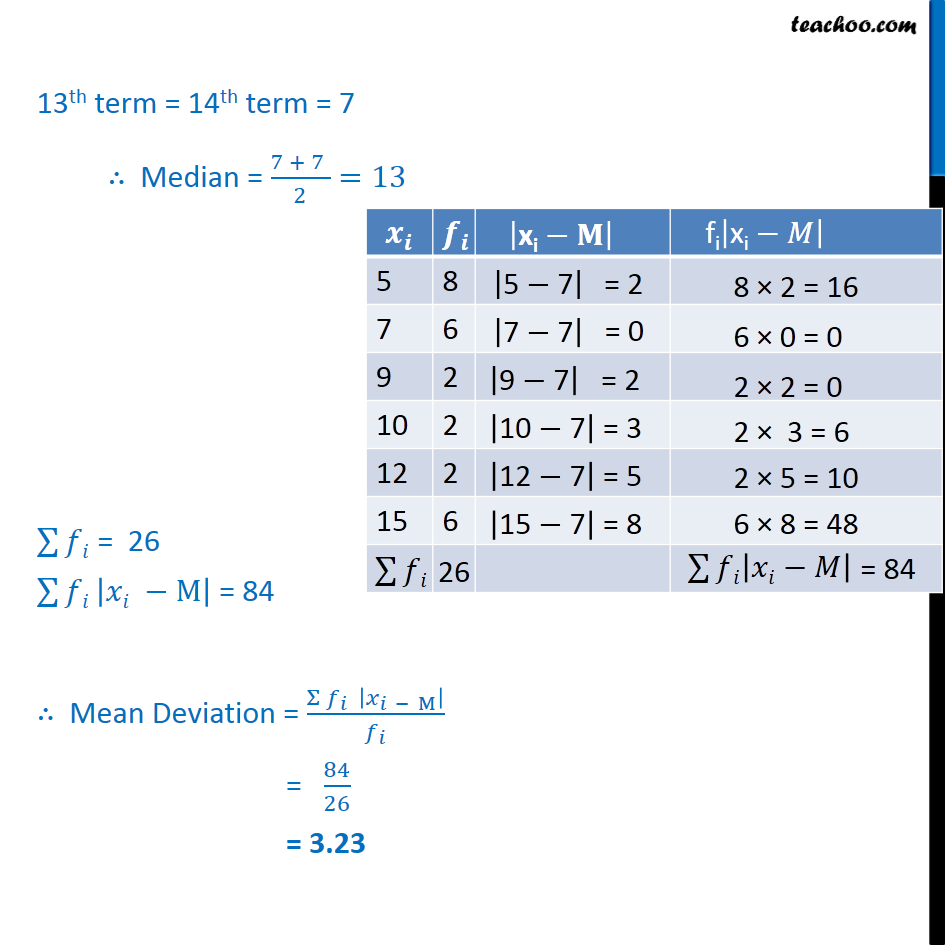Ex 13.1

Chapter 13 Class 11 Statistics
Serial order wiseLearn in your speed, with individual attention - Teachoo Maths 1-on-1 Class

### Transcript

Ex15.1, 7 Find the mean deviation about the median for the data. The given observations are already in ascending order.Adding a column corresponding to cumulative frequencies of the given data, we obtain the following table. c.f = cumulative frequency N = 26 (even) Median = (( /2)^ +( /2 + 1)^ )/2 = ((26/2)^ + (26/2 +1)^ )/2 = ( 13 ^ + 14 ^ )/2 As both observations lie in the c.f of 14 13th term = 14th term = 7 Median = (7 + 7 )/2=13 128 = 26 128 | M| = 84 Mean Deviation = ( _ | _( M) |)/ _ = 84/26 = 3.23